Learn all Concepts of Polynomials Class 9 (with VIDEOS). Check - Polynomials Class 9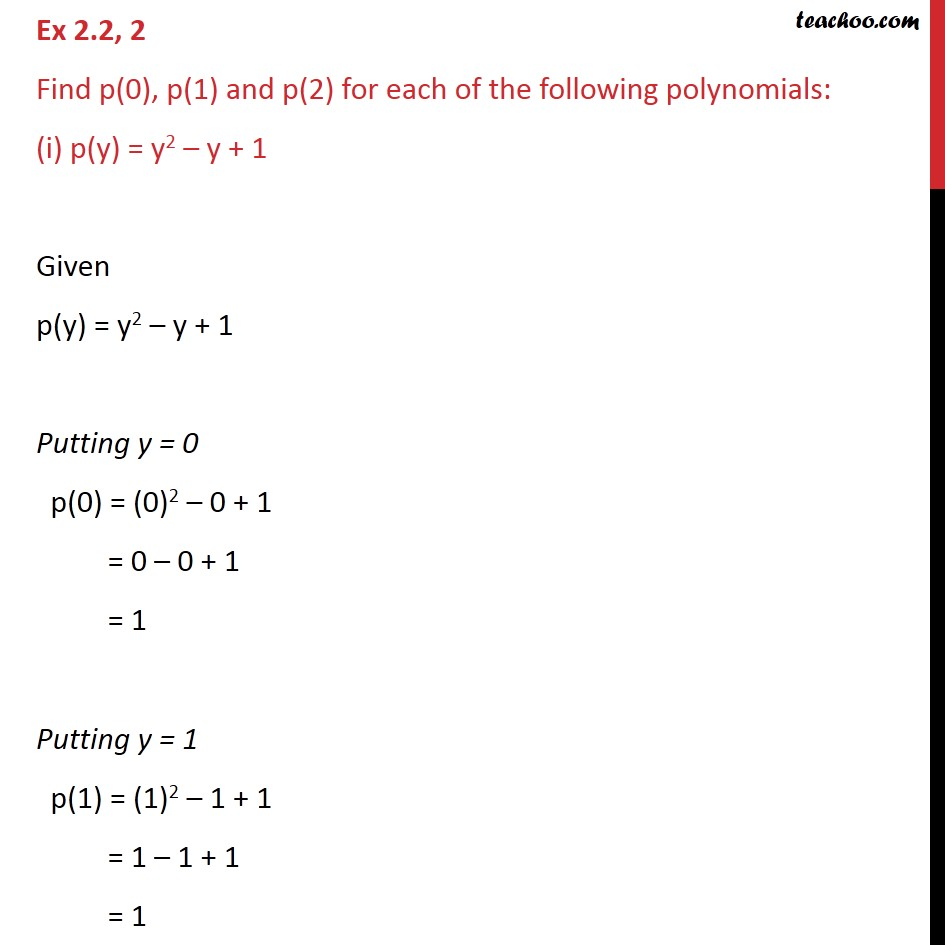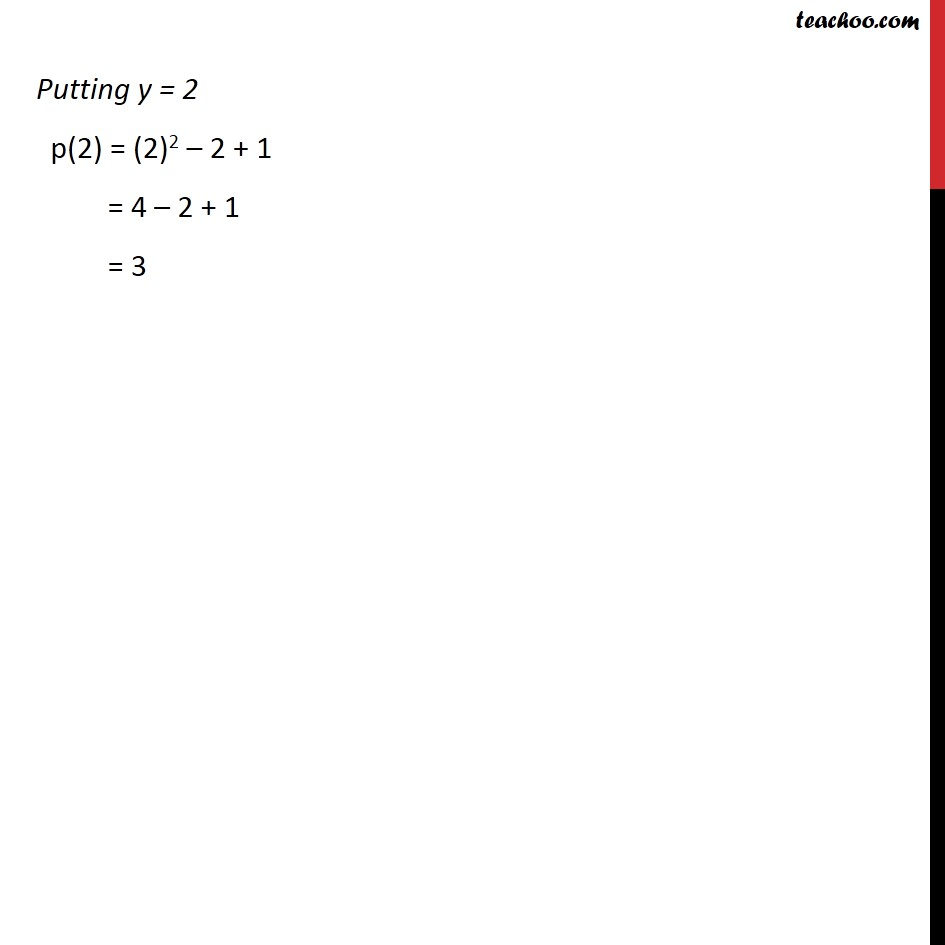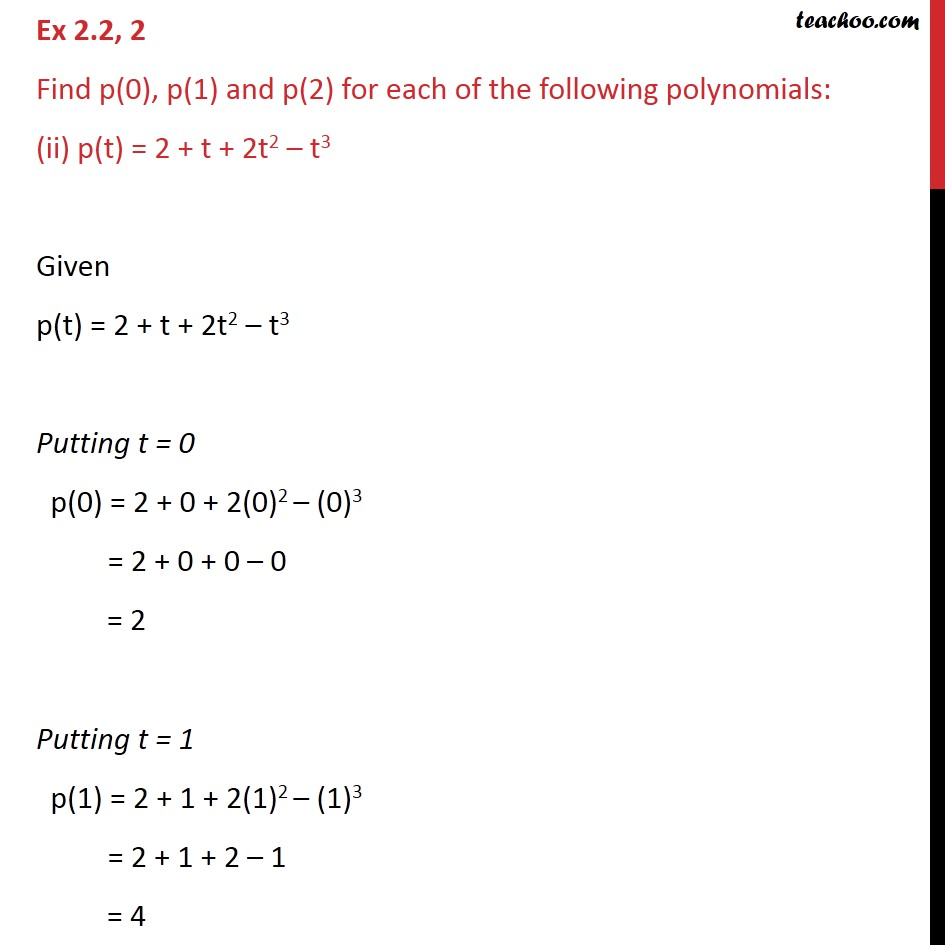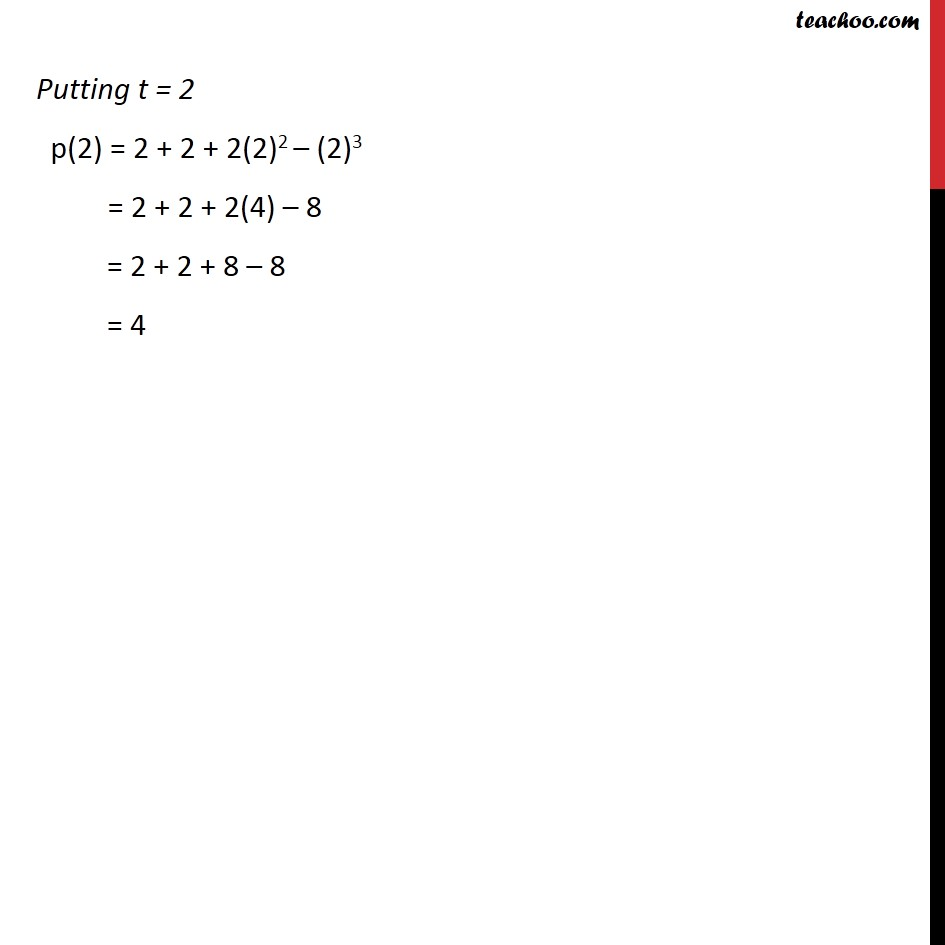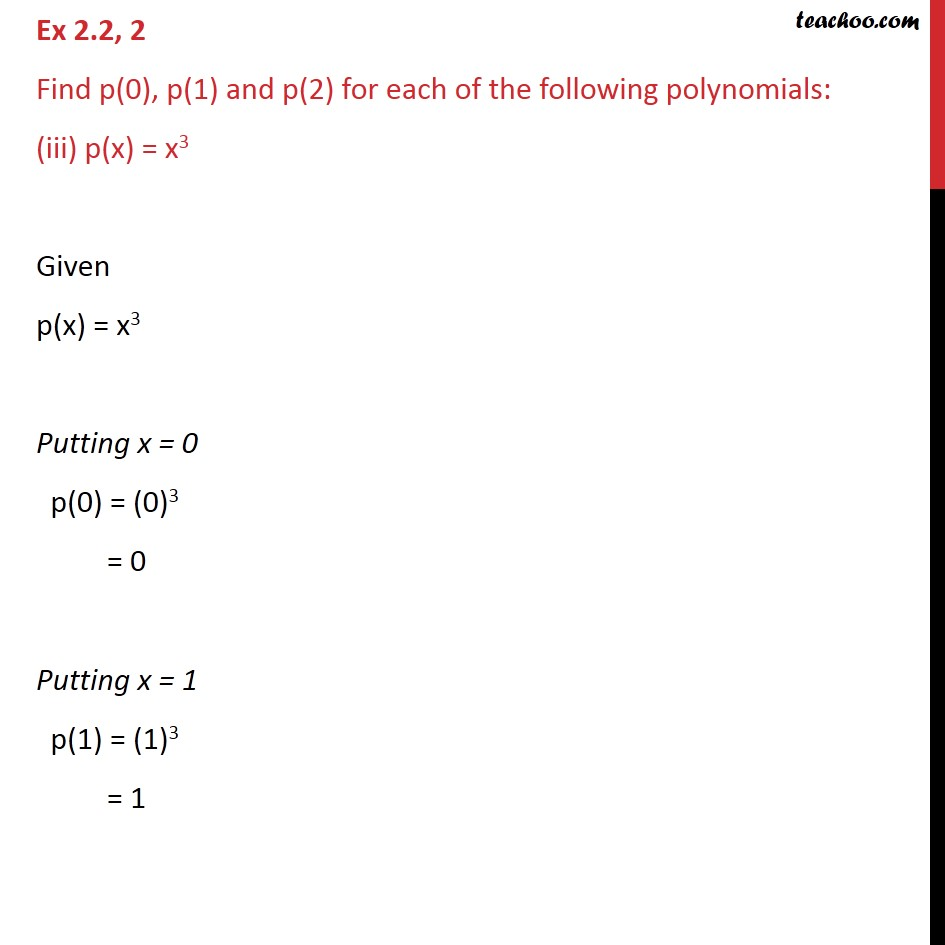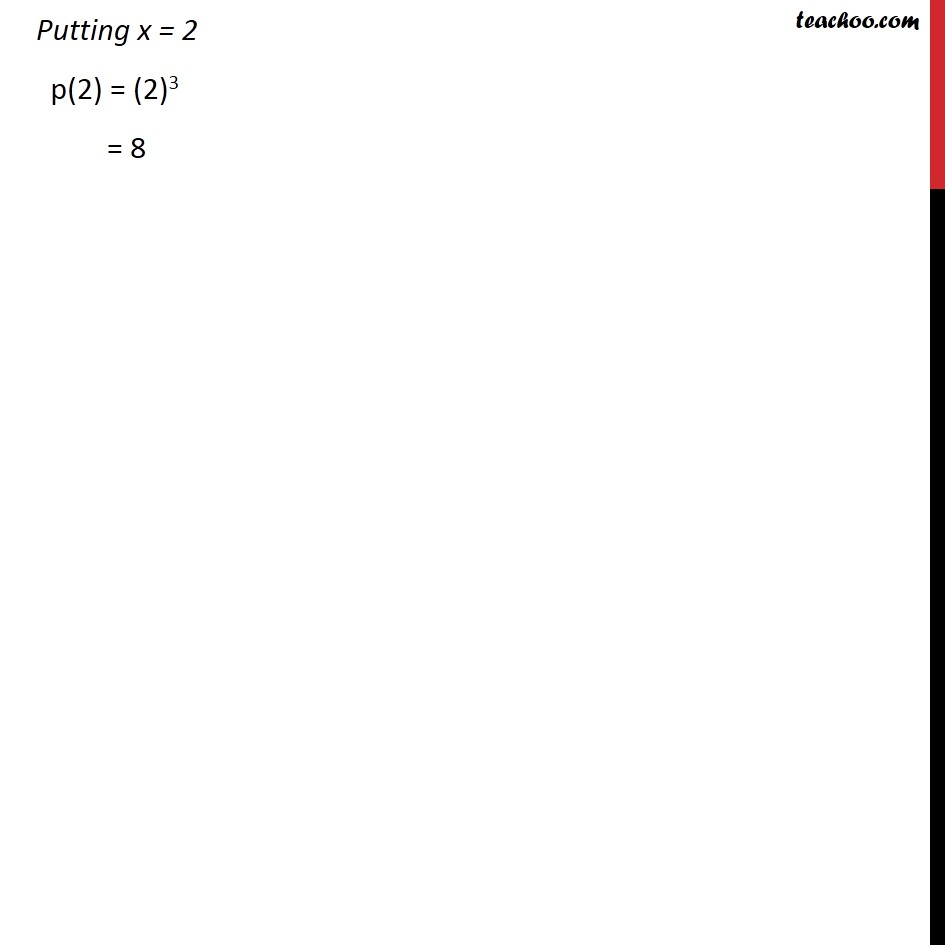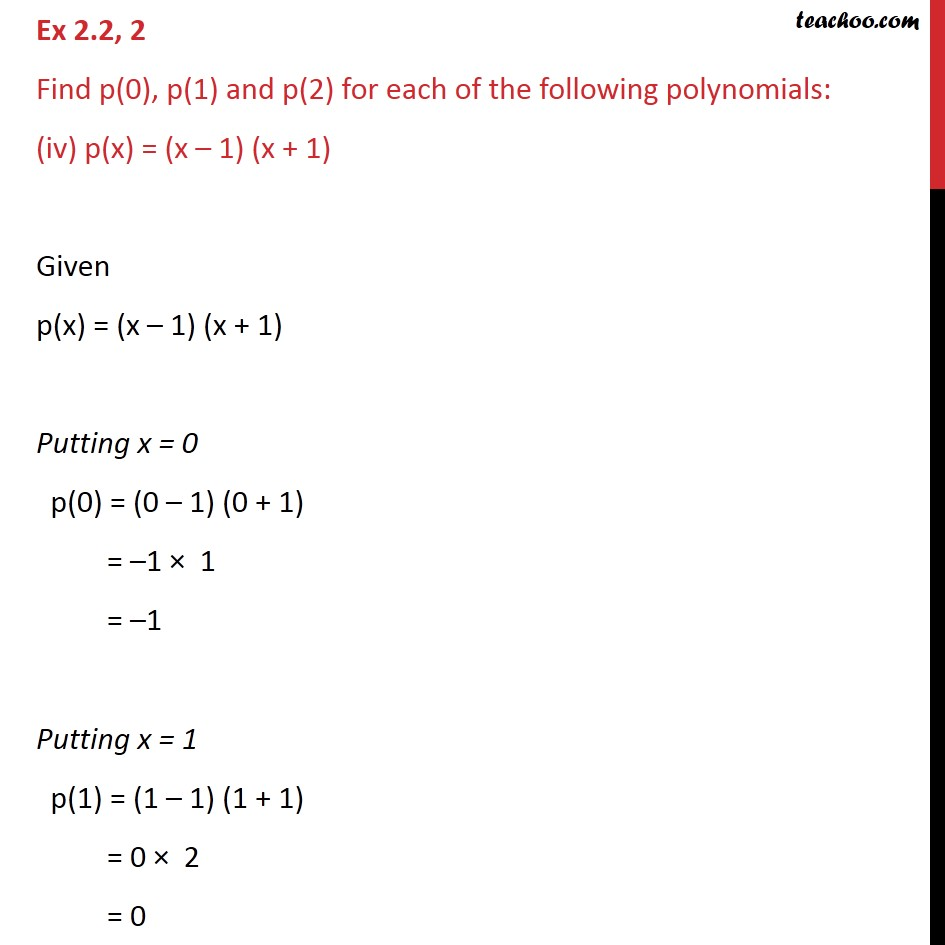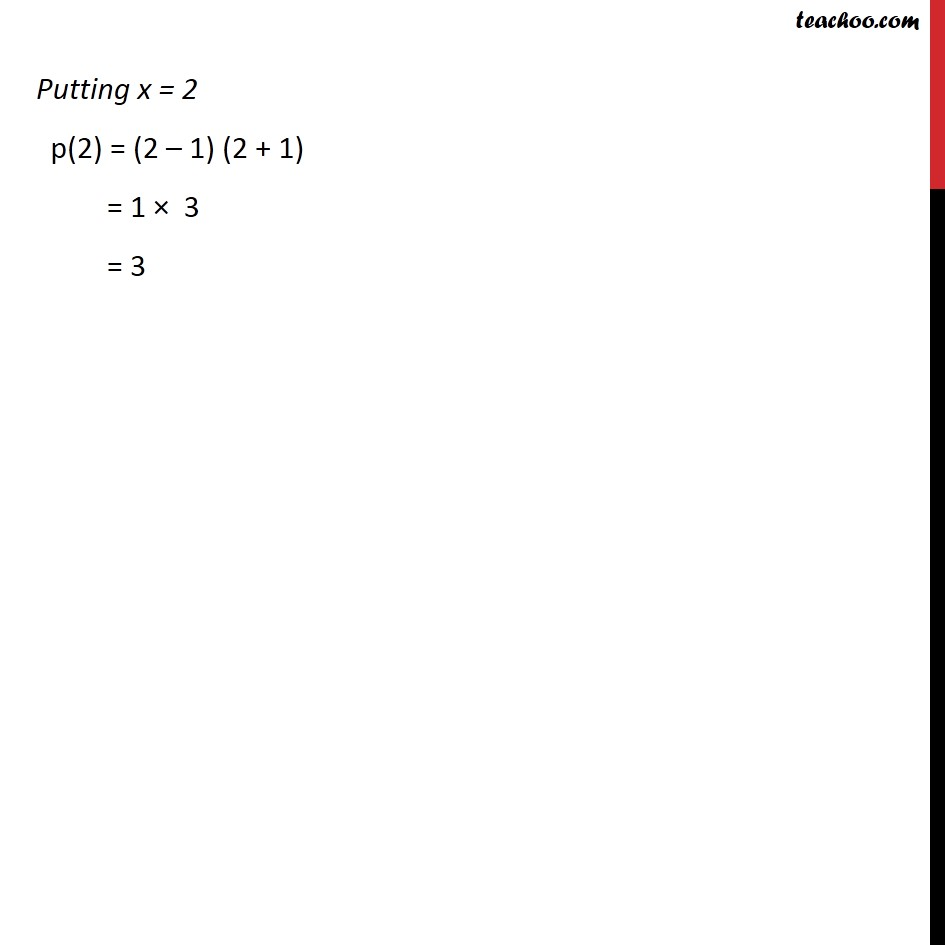1. Chapter 2 Class 9 Polynomials
2. Serial order wise
3. Ex 2.2

Transcript

Ex 2.2, 2 Find p(0), p(1) and p(2) for each of the following polynomials: (i) p(y) = y2 – y + 1 Given p(y) = y2 – y + 1 Putting y = 0 p(0) = (0)2 – 0 + 1 = 0 – 0 + 1 = 1 Putting y = 1 p(1) = (1)2 – 1 + 1 = 1 – 1 + 1 = 1 Putting y = 2 p(2) = (2)2 – 2 + 1 = 4 – 2 + 1 = 3 Ex 2.2, 2 Find p(0), p(1) and p(2) for each of the following polynomials: (ii) p(t) = 2 + t + 2t2 – t3 Given p(t) = 2 + t + 2t2 – t3 Putting t = 0 p(0) = 2 + 0 + 2(0)2 – (0)3 = 2 + 0 + 0 – 0 = 2 Putting t = 1 p(1) = 2 + 1 + 2(1)2 – (1)3 = 2 + 1 + 2 – 1 = 4 Putting t = 2 p(2) = 2 + 2 + 2(2)2 – (2)3 = 2 + 2 + 2(4) – 8 = 2 + 2 + 8 – 8 = 4 Ex 2.2, 2 Find p(0), p(1) and p(2) for each of the following polynomials: (iii) p(x) = x3 Given p(x) = x3 Putting x = 0 p(0) = (0)3 = 0 Putting x = 1 p(1) = (1)3 = 1 Putting x = 2 p(2) = (2)3 = 8 Ex 2.2, 2 Find p(0), p(1) and p(2) for each of the following polynomials: (iv) p(x) = (x – 1) (x + 1) Given p(x) = (x – 1) (x + 1) Putting x = 0 p(0) = (0 – 1) (0 + 1) = –1 × 1 = –1 Putting x = 1 p(1) = (1 – 1) (1 + 1) = 0 × 2 = 0 Putting x = 2 p(2) = (2 – 1) (2 + 1) = 1 × 3 = 3

Ex 2.2

Chapter 2 Class 9 Polynomials
Serial order wise